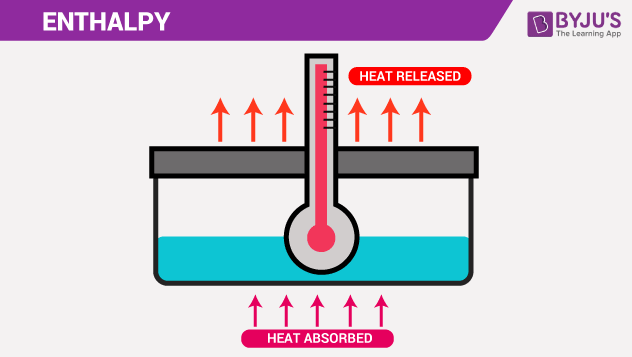# EnthalpyEnthalpy is significant because it tells us how much heat is there in a system (energy). Heat is essential because it allows us to produce useful work. In this article, let’s study Enthalpy.

## What is Enthalpy?

The heat absorbed or released during a process at constant pressure is equal to the change in enthalpy.
Sometimes referred to as “heat content,” enthalpy is a fascinating and uncommon word that most people prefer to use.
According to its Etymology, the words “entropy” and “enthalpy” are derivations of the Greek words for “turning” and “warming,” respectively.
In terms of pronunciation, the first syllable of entropy is commonly stressed, whereas the later syllable of enthalpy is generally emphasised.

Enthalpy(H) is the sum of the internal energy(U) and the product of pressure(P) and volume(V).

Enthalpy H can be written as,

H = U + pV

Where, H = Enthalpy of the system

U = Internal energy of the system

p = Pressure of the system

V = Volume of the system

The heat added or lost by the system is measured as the change in enthalpy (ΔH), not the actual amount of heat. It is totally reliant on T, p, and U, the state functions.
Enthalpy can also be written as:

• ΔH = ΔU + ΔPV

At constant temperature, the heat flow(q) for the process is equal to the change in enthalpy, which is expressed as

• Where ΔH = q (Heat Flow)

### Enthalpy Units

Dimensions can be used to represent any physical quantity. Units are the arbitrary magnitudes assigned to the dimensions. Dimensions are classified into two types: primary or fundamental dimensions and secondary or derived dimensions.

• Primary dimensions:
• Example: Mass (m); length(l); time(t); temperature(T)
• Secondary dimensions:
• Those which can be deduced from primary dimensions.
• Example: Velocity(m/s2), Pressure (Pa = kg/m.s2).
• The Enthalpy is written as,
• H = Energy/Mass
• The secondary physical quantity is enthalpy
• SI Unit of Enthalpy: Joule
• Dimensional Formula: [ML2T-2]

### SI Prefixes:

 Factor Name symbol 1012 Tera T 109 Giga G 106 Mega M 103 Kilo k 102 Hecto h 10-2 Centi c 10-3 Milli m 10-6 Micro $$\begin{array}{l}\mu\end{array}$$ 10-9 Nano n 10-12 Pico p

## What is Thermodynamics?

Thermodynamics is the discipline of physics that studies the interactions of heat and work between a system and its surroundings. It shows how thermal energy interacts with materials and turns into different types of energy.

The first law of thermodynamics is the notion of energy conservation, which is one of nature’s most fundamental rules. It basically states that during an interaction, energy can change forms, but the total amount of energy remains constant.

The second law of thermodynamics states that energy has both quality and quantity and that actual processes occur in the direction of lowering energy quality.

Thermodynamics is involved whenever there is an interaction between energy and matter. Heating and air conditioning systems, refrigerators, water heaters, and other appliances are examples of where thermodynamic law is applicable.## Frequently Asked Questions – FAQs

Q1

### What does thermodynamics mean?

The science of thermodynamics examines how heat, temperature, work, and energy are related. Understanding how an energy level changes inside a system and how the system affects its surroundings is useful.
Q2

### What exactly does enthalpy mean?

The product of pressure and volume is used to calculate enthalpy, which is the total amount of internal energy.
Q3

### What exactly is the first thermodynamics law?

There are two ways to describe thermodynamics:
1. The net heat interaction for the cyclic process will be equal to the network interaction.
2. According to the first rule of thermodynamics: for a certain process, net heat energy is the total of internal energy change and work interaction.
Q4

### What exactly is the second thermodynamics law?

The second law says that the total entropy of an interacting system never decreases in a natural thermodynamic occurrence. In another way, heat does not transfer spontaneously from a colder system to a warmer system.
Q5

### What is the third law of thermodynamics?

The third law states that when the temperature approaches absolute zero, a system’s entropy will begin to approach a fixed value. At absolute zero, a system’s entropy is often near zero, with the exception of non-crystalline materials.
Learn more about enthalpy, laws of thermodynamics and a lot more. At BYJU’S we also provide free CBSE  Sample Papers and Study Notes for a better understanding of the topics.